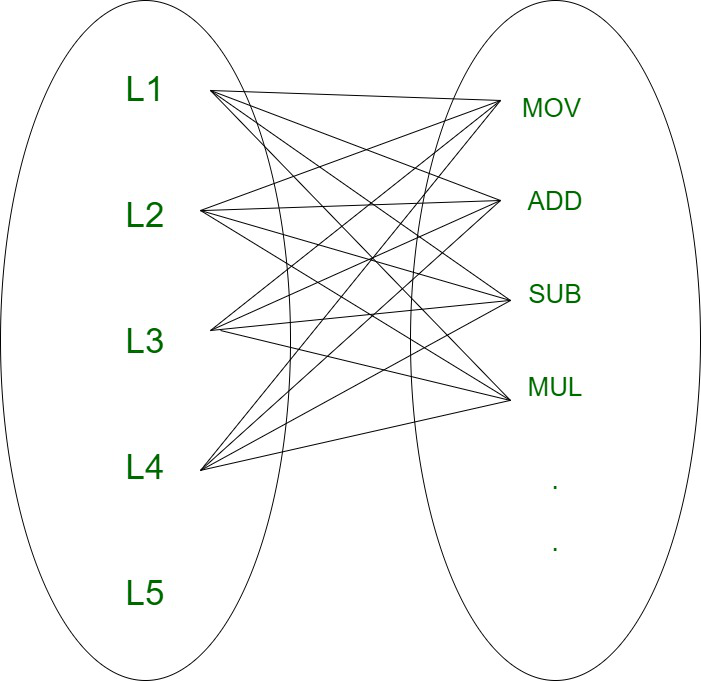# Target Code Generation in Compiler Design

Target code generation is the final Phase of Compiler.

1. Input : Optimized Intermediate Representation.
2. Output : Target Code.
3. Task Performed : Register allocation methods and optimization, assembly level code.
4. Method : Three popular strategies for register allocation and optimization.
5. Implementation : Algorithms.
6. Target code generation deals with assembly language to convert optimized code into machine understandable format. Target code can be machine readable code or assembly code. Each line in optimized code may map to one or more lines in machine (or) assembly code, hence there is a 1:N mapping associated with them .1 : N Mapping

Computations are generally assumed to be performed on high speed memory locations, known as registers. Performing various operations on registers is efficient as registers are faster than cache memory. This feature is effectively used by compilers, However registers are not available in large amount and they are costly. Therefore we should try to use minimum number of registers to incur overall low cost.

Optimized code :

```Example 1 :
L1: a = b + c * d

optimization :
t0 = c * d
a  = b + t0
Example 2 :
L2: e = f - g / d

optimization :
t0 = g / d
e  = f - t0
```

Register Allocation :
Register allocation is the process of assigning program variables to registers and reducing the number of swaps in and out of the registers. Movement of variables across memory is time consuming and this is the main reason why registers are used as they available within the memory and they are the fastest accessible storage location.

```Example 1:
R1<--- a
R2<--- b
R3<--- c
R4<--- d

MOV R3, c
MOV R4, d
MUL R3, R4
MOV R2, b
MOV R1, R2
MOV a, R1
Example 2:
R1<--- e
R2<--- f
R3<--- g
R4<--- h

MOV R3, g
MOV R4, h
DIV R3, R4
MOV R2, f
SUB R2, R3
MOV R1, R2
MOV e, R1 ```

• Fast accessible storage
• Allows computations to be performed on them
• Deterministic as it incurs no miss
• Reduce memory traffic
• Reduces overall computation time